Singular Matrices: Zero Determinants

When determinants are zero

Create a matrix with one row as a linear combination of the others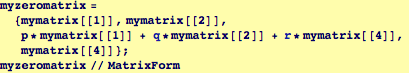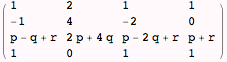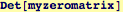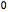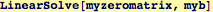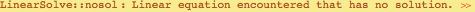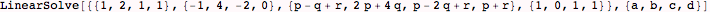This was not expected to have a solution because one of four equations in the system was a linear combination of others in the system. Effectively, we were asking Mathematica to solve a system of three equations in four unknowns. The rank of a square matrix of coefficients is equal to the number of linearly independent equations in the system. The null space of the matrix will be empty when the equations are all linearly independent.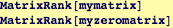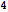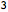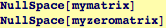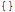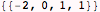Try solving this inhomogeneous system of equations using Solve: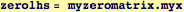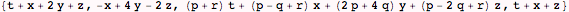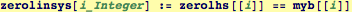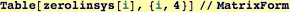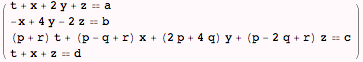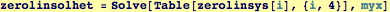No solution, as expected,  Let's see what happens if we ask Mathematica to solve the homogeneous problem: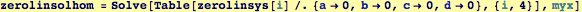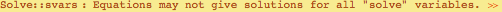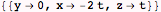In this case, Mathematica gives a relationship between the variables, but because there are fewer equations than variables, there is still no unique solution.

 Created by Wolfram Mathematica 6.0  (06 September 2007)